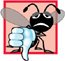# E.3. Variable-Length Argument Lists

### E 3 Variable Length Argument Lists

 In C++, programmers use function overloading to accomplish much of what C programmers accomplish with variable-length argument lists.

It is possible to create functions that receive an unspecified number of arguments. An ellipsis (...) in a function's prototype indicates that the function receives a variable number of arguments of any type. Note that the ellipsis must always be placed at the end of the parameter list, and there must be at least one argument before the ellipsis. The macros and definitions of the variable arguments header (Fig. E.1) provide the capabilities necessary to build functions with variable-length argument lists.

Figure E.1. The type and the macros defined in header .

(This item is displayed on page 1250 in the print version)

Identifier

Description

va_list

A type suitable for holding information needed by macros va_start, va_arg and va_end. To access the arguments in a variable-length argument list, an object of type va_list must be declared.

va_start

A macro that is invoked before the arguments of a variable-length argument list can be accessed. The macro initializes the object declared with va_list for use by the va_arg and va_end macros.

va_arg

A macro that expands to an expression of the value and type of the next argument in the variable-length argument list. Each invocation of va_arg modifies the object declared with va_list so that the object points to the next argument in the list.

va_end

A macro that performs termination housekeeping in a function whose variable-length argument list was referred to by the va_start macro.

Figure E.2 demonstrates function average that receives a variable number of arguments. The first argument of average is always the number of values to be averaged, and the remainder of the arguments must all be of type double.

Figure E.2. Using variable-length argument lists.

(This item is displayed on pages 1250 - 1251 in the print version)

``` 1 // Fig. E.2: figE_02.cpp
2 // Using variable-length argument lists.
3 #include
4 using std::cout;
5 using std::endl;
6 using std::ios;
7
8 #include
9 using std::setw;
10 using std::setprecision;
11 using std::setiosflags;
12 using std::fixed;
13
14 #include
15 using std::va_list;
16
17 double average( int, ... );
18
19 int main()
20 {
21 double double1 = 37.5;
22 double double2 = 22.5;
23 double double3 = 1.7;
24 double double4 = 10.2;
25
26 cout << fixed << setprecision( 1 ) << "double1 = "
27 << double1 << "
double2 = " << double2 << "
double3 = "
28 << double3 << "
double4 = " << double4 << endl
29 << setprecision( 3 )
30 << "
The average of double1 and double2 is "
31 << average( 2, double1, double2 )
32 << "
The average of double1, double2, and double3 is "
33 << average( 3, double1, double2, double3 )
34 << "
The average of double1, double2, double3"
35 << " and double4 is "
36 << average( 4, double1, double2, double3, double4 )
37 << endl;
38 return 0;
39 } // end main
40
41 // calculate average
42 double average( int count, ... )
43 {
44 double total = 0;
45 va_list list; // for storing information needed by va_start
46
47 va_start( list, count );
48
49 // process variable-length argument list
50 for ( int i = 1; i <= count; i++ )
51 total += va_arg( list, double );
52
53 va_end( list ); // end the va_start
55 } // end function average
```

 ``` double1 = 37.5 double2 = 22.5 double3 = 1.7 double4 = 10.2 The average of double1 and double2 is 30.000 The average of double1, double2, and double3 is 20.567 The average of double1, double2, double3 and double4 is 17.975 ```

Function average uses all the definitions and macros of header . Object list, of type va_list, is used by macros va_start, va_arg and va_end to process the variable-length argument list of function average. The function invokes va_start to initialize object list for use in va_arg and va_end. The macro receives two argumentsobject list and the identifier of the rightmost argument in the argument list before the ellipsiscount in this case (va_start uses count here to determine where the variable-length argument list begins).

Next, function average repeatedly adds the arguments in the variable-length argument list to the total. The value to be added to total is retrieved from the argument list by invoking macro va_arg. Macro va_arg receives two argumentsobject list and the type of the value expected in the argument list (double in this case)and returns the value of the argument. Function average invokes macro va_end with object list as an argument before returning. Finally, the average is calculated and returned to main. Note that we used only double arguments for the variable-length portion of the argument list.

Variable-length argument lists promote variables of type float to type double. These argument lists also promote integral variables that are smaller than int to type int (variables of type int, unsigned, long and unsigned long are left alone).

Software Engineering Observation E.1Variable-length argument lists can be used only with fundamental type arguments and with arguments of C-style struct types that do not contain C++ specific features such as virtual functions, constructors, destructors, references, const data members and virtual base classes.

Common Programming Error E.1Placing an ellipsis in the middle of a function parameter list is a syntax error. An ellipsis may be placed only at the end of the parameter list.C++ How to Program (5th Edition)
ISBN: 0131857576
EAN: 2147483647
Year: 2004
Pages: 627